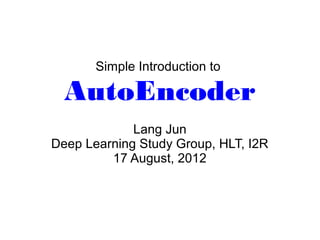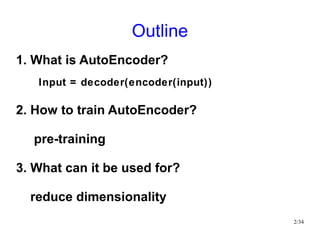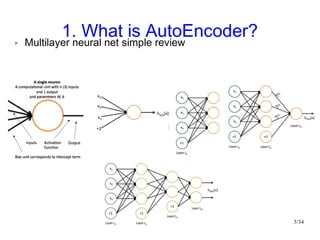Successfully reported this slideshow.

# Simple Introduction to AutoEncoder

64

Share×
1 of 35
1 of 35

# Simple Introduction to AutoEncoder

64

Share

Simple Introduction to AutoEncoder

Simple Introduction to AutoEncoder

## More Related Content

### Related Books

Free with a 14 day trial from Scribd

See all

### Related Audiobooks

Free with a 14 day trial from Scribd

See all

### Simple Introduction to AutoEncoder

1. 1. Simple Introduction to AutoEncoder Lang Jun Deep Learning Study Group, HLT, I2R 17 August, 2012
2. 2. Outline 1. What is AutoEncoder? Input = decoder(encoder(input)) 2. How to train AutoEncoder? pre-training 3. What can it be used for? reduce dimensionality 2/34
3. 3. 1. What is AutoEncoder? ➢ Multilayer neural net simple review 3/34
4. 4. 1. What is AutoEncoder? ➢ Multilayer neural net simple review 4/34
5. 5. 1. What is AutoEncoder? ➢ Multilayer neural net simple review 5/34
6. 6. 1. What is AutoEncoder? ➢ Multilayer neural net simple review 6/34
7. 7. 1. What is AutoEncoder? ➢ Multilayer neural net simple review 7/34
8. 8. 1. What is AutoEncoder? ➢ Multilayer neural net simple review 8/34
9. 9. 1. What is AutoEncoder? ➢ Multilayer neural net simple review 9/34
10. 10. 1. What is AutoEncoder? ➢ Multilayer neural net simple review 10/34
11. 11. 1. What is AutoEncoder? ➢ Multilayer neural net simple review 11/34
12. 12. 1. What is AutoEncoder? ➢ Multilayer neural net simple review 12/34
13. 13. 1. What is AutoEncoder? ➢ Multilayer neural net simple review 13/34
14. 14. 1. What is AutoEncoder? ➢ Multilayer neural net simple review 14/34
15. 15. 1. What is AutoEncoder? ➢ Multilayer neural net simple review 15/34
16. 16. 1. What is AutoEncoder? ➢ Multilayer neural net simple review 16/34
17. 17. 1. What is AutoEncoder? ➢ Multilayer neural net simple review 17/34
18. 18. 1. What is AutoEncoder? ➢ Multilayer neural net simple review 18/34
19. 19. 1. What is AutoEncoder? ➢ Multilayer neural net simple review 19/34
20. 20. 1. What is AutoEncoder? ➢ Multilayer neural net simple review 20/34
21. 21. 1. What is AutoEncoder? ➢ Multilayer neural net simple review 21/34
22. 22. 1. What is AutoEncoder? ➢ Multilayer neural net simple review 22/34
23. 23. 1. What is AutoEncoder? ➢ Multilayer neural net simple review 23/34
24. 24. 1. What is AutoEncoder? ➢ Multilayer neural net with target output = input ➢ Reconstruction=decoder(encoder(input)) ➢ Minimizing reconstruction error ➢ Probable inputs have small reconstruction error 24/34
25. 25. 2. How to train AutoEncoder? Hinton (2006) Science Paper Restricted Boltzmann Machine (RBM) 25/34
26. 26. 2. How to train AutoEncoder? Hinton (2006) Science Paper restricted Boltzmann machine 26/34
27. 27. Effective deep learning became possible through unsupervised pre- training Purely supervised neural net With unsupervised pre‐training (with RBMs and Denoising Auto-Encoders) 27/34 0–9 handwritten digit recognition error rate (MNIST data)
28. 28. Why is unsupervised pre-training working so well? Regularization hypothesis: Representations good for P(x) are good for P(y|x) Optimization hypothesis: Unsupervised initializations start near better local minimum of supervised training error Minima otherwise not achievable by random initialization Erhan, Courville, Manzagol, Vincent, Bengio (JMLR, 2010) 28/34
29. 29. 3. What can it be used for? illustration for images 29/34
30. 30. 3. What can it be used for? document retrieval output 2000 reconstructed counts vector • We train the neural network 500 neurons to reproduce its input vector as its output • This forces it to compress as 250 neurons much information as possible into the 10 numbers in the central bottleneck. 10 • These 10 numbers are then a good way to compare documents. 250 neurons – See Ruslan Salakhutdinov’s talk 500 neurons input 30/34 2000 word counts vector
31. 31. 3. What can it be used for? visualize documents output 2000 reconstructed counts vector • Instead of using codes to retrieve documents, we can 500 neurons use 2-D codes to visualize sets of documents. – This works much better 250 neurons than 2-D PCA 2 250 neurons 500 neurons input 31/34 2000 word counts vector
32. 32. First compress all documents to 2 numbers using a type of PCA Then use different colors for different document categories 32/34
33. 33. First compress all documents to 2 numbers with an autoencoder Then use different colors for different document categories 33/34
34. 34. 3. What can it be used for? transliteration 34/34
35. 35. Thanks for your attendance Looking forward to present Recursive AutoEncoder 35/34